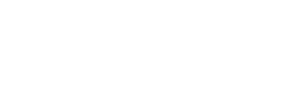kg

### 46AT5/14-2 Aluminium Pulley

• Belt Width                             =  32mm
• Length Through Bore  mm  =  46mm
• Pitch mm  AT5                        =  5mm
• Number Teeth                      =  14
• Diameter over teeth mm     =  21.05
• Material  / Bore                    =  Aluminium Pilot Bore
210701406
kg

### 46AT5/15-2 Aluminium Pulley

• Belt Width                             =  32mm
• Length Through Bore  mm  =  46mm
• Pitch mm  AT5                        =  5mm
• Number Teeth                      =  15
• Diameter over teeth mm     =  22.65
• Material  / Bore                    =  Aluminium Pilot Bore
210701506
kg

### 46AT5/16-2 Aluminium Pulley

• Belt Width                             =  32mm
• Length Through Bore  mm  =  46mm
• Pitch mm  AT5                        =  5mm
• Number Teeth                      =  16
• Diameter over teeth mm     =  24.20
• Material  / Bore                    =  Aluminium Pilot Bore
210701606
kg

### 46AT5/18-2 Aluminium Pulley

• Belt Width                             =  32mm
• Length Through Bore  mm  =  46mm
• Pitch mm  AT5                        =  5mm
• Number Teeth                      =  18
• Diameter over teeth mm     =  27.40
• Material  / Bore                    =  Aluminium Pilot Bore
210701806
kg

### 46AT5/19-2 Aluminium Pulley

• Belt Width                             =  32mm
• Length Through Bore  mm  =  46mm
• Pitch mm  AT5                        =  5mm
• Number Teeth                      =  19
• Diameter over teeth mm     =  29.00
• Material  / Bore                    =  Aluminium Pilot Bore
210701906
kg

### 46AT5/20-2 Aluminium Pulley

• Belt Width                             =  32mm
• Length Through Bore  mm  =  46mm
• Pitch mm  AT5                        =  5mm
• Number Teeth                      =  20
• Diameter over teeth mm     =  30.60
• Material  / Bore                    =  Aluminium Pilot Bore
210702006
kg

### 46AT5/22-2 Aluminium Pulley

• Belt Width                             =  32mm
• Length Through Bore  mm  =  46mm
• Pitch mm  AT5                        =  5mm
• Number Teeth                      =  22
• Diameter over teeth mm     =  33.85
• Material  / Bore                    =  Aluminium Pilot Bore
210702206
kg

### 46AT5/24-2 Aluminium Pulley

• Belt Width                             =  32mm
• Length Through Bore  mm  =  46mm
• Pitch mm  AT5                        =  5mm
• Number Teeth                      =  24
• Diameter over teeth mm     =  37.00
• Material  / Bore                    =  Aluminium Pilot Bore
210702406
kg

### 46AT5/25-2 Aluminium Pulley

• Belt Width                             =  32mm
• Length Through Bore  mm  =  46mm
• Pitch mm  AT5                        =  5mm
• Number Teeth                      =  25
• Diameter over teeth mm     =  38.60
• Material  / Bore                    =  Aluminium Pilot Bore
210702506
kg

### 46AT5/26-2 Aluminium Pulley

• Belt Width                             =  32mm
• Length Through Bore  mm  =  46mm
• Pitch mm  AT5                        =  5mm
• Number Teeth                      =  26
• Diameter over teeth mm     =  40.20
• Material  / Bore                    =  Aluminium Pilot Bore
210702606
kg

### 46AT5/27-2 Aluminium Pulley

• Belt Width                             =  32mm
• Length Through Bore  mm  =  46mm
• Pitch mm  AT5                        =  5mm
• Number Teeth                      =  27
• Diameter over teeth mm     =  41.80
• Material  / Bore                    =  Aluminium Pilot Bore
210702706
kg

### 46AT5/28-2 Aluminium Pulley

• Belt Width                             =  32mm
• Length Through Bore  mm  =  46mm
• Pitch mm  AT5                        =  5mm
• Number Teeth                      =  28
• Diameter over teeth mm     =  43.35
• Material  / Bore                    =  Aluminium Pilot Bore
210702806
kg

### 46AT5/30-2 Aluminium Pulley

• Belt Width                             =  32mm
• Length Through Bore  mm  =  46mm
• Pitch mm  AT5                        =  5mm
• Number Teeth                      =  30
• Diameter over teeth mm     =  46.55
• Material  / Bore                    =  Aluminium Pilot Bore
210703006
kg

### 46AT5/32-2 Aluminium Pulley

• Belt Width                             =  32mm
• Length Through Bore  mm  =  46mm
• Pitch mm  AT5                        =  5mm
• Number Teeth                      =  32
• Diameter over teeth mm     =  49.70
• Material  / Bore                    =  Aluminium Pilot Bore
210703206
kg

### 46AT5/36-2 Aluminium Pulley

• Belt Width                             =  32mm
• Length Through Bore  mm  =  46mm
• Pitch mm  AT5                        =  5mm
• Number Teeth                      =  36
• Diameter over teeth mm     =  56.05
• Material  / Bore                    =  Aluminium Pilot Bore
210703606
kg

### 46AT5/40-2 Aluminium Pulley

• Belt Width                             =  32mm
• Length Through Bore  mm  =  46mm
• Pitch mm  AT5                        =  5mm
• Number Teeth                      =  40
• Diameter over teeth mm     =  62.45
• Material  / Bore                    =  Aluminium Pilot Bore
210704006
kg

### 46AT5/48-2 Aluminium Pulley

• Belt Width                             =  32mm
• Length Through Bore  mm  =  46mm
• Pitch mm  AT5                        =  5mm
• Number Teeth                      =  48
• Diameter over teeth mm     =  75.15
• Material  / Bore                    =  Aluminium Pilot Bore
210704806